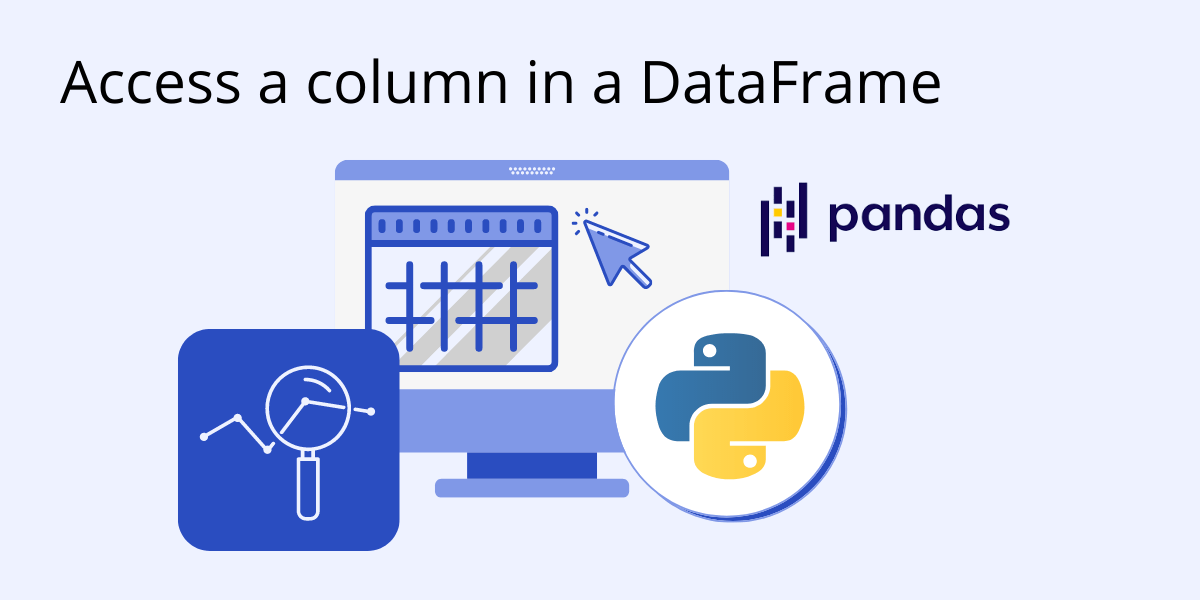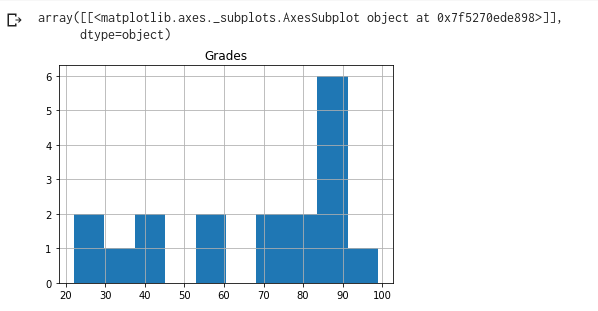# How to Access a Column in a DataFrameBefore we start: This Python tutorial is a part of our series of Python Package tutorials. The steps explained ahead are related to the sample project introduced here.
You can use the loc and iloc functions to access columns in a Pandas DataFrame. Let’s see how.

We will first read in our CSV file by running the following line of code:

`Report_Card = pd.read_csv("Report_Card.csv")`

This will provide us with a DataFrame that looks like the following:If we wanted to access a certain column in our DataFrame, for example the Grades column, we could simply use the loc function and specify the name of the column in order to retrieve it.

`Report_Card.loc[:,"Grades"]`The first argument ( : ) signifies which rows we would like to index, and the second argument (Grades) lets us index the column we want. The semicolon returns all of the rows from the column we specified.

The same result can also be obtained using the iloc function. iloc arguments require integer-value indices instead of string-value names. To reproduce our Grades column example we can use the following code snippet:

`Report_Card.iloc[:,3]`

Since the Name column is the 0’th column, the Grades column will have the numerical index value of 3.

We can also access multiple columns at once using the loc function by providing an array of arguments, as follows:

`Report_Card.loc[:,["Lectures","Grades"]]`

To obtain the same result with the iloc function we would provide an array of integers for the second argument.

`Report_Card.iloc[:,[2,3]]`

Both the iloc and loc function examples will produce the following DataFrame:It is important to note that the order of the column names we used when specifying the array affects the order of the columns in the resulting DataFrame, as can be seen in the above image.

Cleaning Data

When cleaning data we will sometimes need to deal with NaNs (Not a Number values). To search for columns that have missing values, we could do the following:

```nans_indices = Report_Card.columns[Report_Card.isna().any()].tolist()
nans = Report_Card.loc[:,nans]```

When we use the Report_Card.isna().any() argument we get a Series Object of boolean values, where the values will be True if the column has any missing data in any of their rows. This Series Object is then used to get the columns of our DataFrame with missing values, and turn it into a list using the tolist() function. Finally we use these indices to get the columns with missing values.

Visualization

Since we now have the column named Grades, we can try to visualize it. Normally we would use another Python package to plot the data, but luckily pandas provides some built-in visualization functions. For example, we can get a histogram of the Grades column using the following line of code:

/* Code Block */

`Grades.hist()`

/* Code Block */

This will produce the following histogram for us, where we can check the distribution of the grades. Since our data is not organic and very limited in numbers, our distribution is also quite unrealistic. Nonetheless here is the histogram:#### Next steps

Now that you know how to access a column in a DataFrame using Python’s Pandas library, let’s move on to other things you can do with Pandas:

## Python For Data Science

Pre-bundled with the most important packages Data Scientists need, ActivePython is pre-compiled so you and your team don’t have to waste time configuring the open source distribution. You can focus on what’s important–spending more time building algorithms and predictive models against your big data sources, and less time on system configuration.

### Some Popular Python Packages for Data Science/Big Data/Machine LearningYou Get Pre-compiled – with ActivePython

• pandas (data analysis)
• NumPy (multi-dimensional arrays)
• SciPy (algorithms to use with numpy)
• HDF5 (store & manipulate data)
• Matplotlib (data visualization)
• Jupyter (research collaboration)
• PyTables (managing HDF5 datasets)
• HDFS (C/C++ wrapper for Hadoop)
• pymongo (MongoDB driver)
• SQLAlchemy (Python SQL Toolkit)
With deep roots in open source, and as a founding member of the Python Foundation, ActiveState actively contributes to the Python community. We offer the convenience, security and support that your enterprise needs while being compatible with the open source distribution of Python.